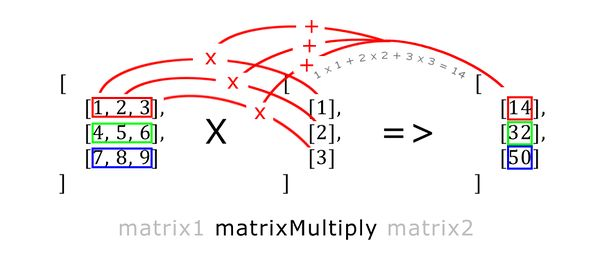# matrixMultiply

Hover & click on the images for description

### Description

Description:
Returns resulting matrix from the multiplication of two matrices. First matrix must be of the size n ✕ k, while the second must be of the size k ✕ m, i.e columns matrix1 == rows matrix2. The resulting matrix will be of the size n ✕ m. If the shapes do not match, empty array [] will be returned.Groups:
MathMath - Vectors

### Syntax

Syntax:
matrix1 matrixMultiply matrix2
Parameters:
matrix1: Array - matrix of the size n(rows)k(columns)
matrix2: Array - matrix of the size k(rows)m(columns)
Return Value:
Array - resulting matrix of the size n(rows)m(columns)

### Examples

Example 1:
```[ ,  ] matrixMultiply [  ] /* returns [ ,  ] */```
Example 2:
```[ [-1,0,0], [0,-1,0] ] matrixMultiply [ [1,2], [3,1], [2,3] ] /* returns [ [-1,-2], [-3,-1] ] */```

Note that this command won't transform a 1D array automatically into a column vector ```[[-1,0,0],[0,-1,0],[0,0,-1]] matrixMultiply [1,2,3] // wrong, syntax error [[-1,0,0],[0,-1,0],[0,0,-1]] matrixMultiply [[1,2,3]] // wrong, will return [] [[-1,0,0],[0,-1,0],[0,0,-1]] matrixMultiply [, , ] // correct, will return [[-1], [-2], [-3]] ```